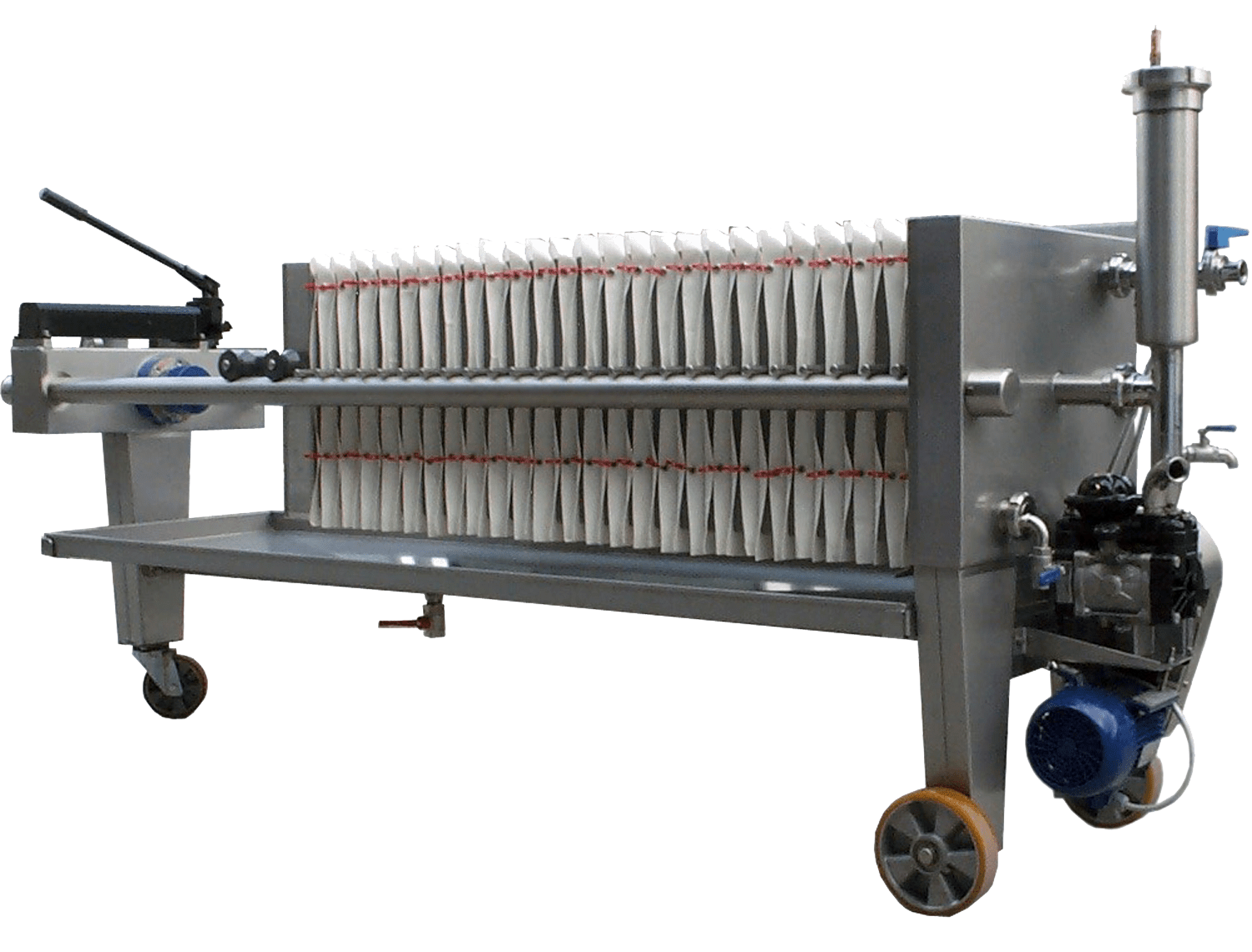# A Simple calculation on How to Determine Time Required to Obtain Certain Volume of Filtrate

In this post, I want to share how to do a simple calculation on determination of time required to obtain certain amount of filtrate in a filtration test. We will still use basic equation as in previous post.

Read previous post: How to Determine Cake Resistance in Filtration Systems

For example, we have this case.

A filter press with a surface of 50 m2

C (weight of solid per volume of liquid) = 135 kg solid/m3 liquid

μ = 0.001 N sec/m2

α (specific resistance of the cake) = 1.1 (1011) m/kg

Rf (resistance of filter cloth) = 6.5 (1010) m-1

The feed pump is a centrifugal with a characteristic curve represented by the equation:

∆P = 2 – Q (0.00163 Q – 0.02889), in bar

With Q in m3/hr.

Calculate:

1. Time required to obtain 50 m3 of filtrate
2. Volume, flow rate, and pressure profiles

Solution:

Using the following equation and combined with pump characteristic curve equation to solve for V (volume of filtrate).$Q = \frac{{dV}}{{dt}} = \frac{{A\Delta P}}{{\mu ({R_f} + {R_C})}} = \frac{{A\Delta P}}{{\mu ({R_f} + \alpha cV/A)}}$

Where:

α = specific resistance of the cake (m/kg)

c = weight of solids per volume of liquid (kg/m3)

μ = viscosity (N sec/m2)

P = pressure difference (N/m2)

A = filtering surface (m2)

V = volume of filtrate (m3)

Q = rate of filtrate accumulation (m3/sec)

Rf and α are constants of the equipment and slurry and must be evaluated from experimental data.

We get the following equation:$V = \left( {\frac{{A\Delta P}}{{Q\mu }} - {R_f}} \right)\frac{A}{{C\alpha }}\\$$V = \left( {\frac{{A\Delta P}}{Q} - {R_f}\mu } \right)\frac{A}{{C\alpha \mu }}\\$$V = \left( {\frac{{50({{10}^5})\Delta P}}{{Q/3600}} - 6.5({{10}^{10}})(0.001)} \right)\frac{{50}}{{10(1.1({{10}^{11}}))(0.001)}}\\$$V = 818\frac{{\Delta P}}{Q} - 3$

Please be noted that the unit of pressure used above is in bar. It should be converted to Pa by multiplying with 10,000. The rate of filtrate accumulation is also in m3/hour. It should be converted to m3/second by multiplying with 1/3,600.

Then we need to have the following set of data: V, ∆P, Q, and t. To get these data, we need to try and error, or we use goal seek in spreadsheet.

1. Determine V. Because the problem is how to determine time to get 50 m3 filtrate, then we can determine V from 0 to 50 m3. For example, 0, 10, 20, 30, 40 and 50 m3.
2. Then, we determine ∆P/Q using equation above. ∆P/Q = (V + 3) / 181. Do this for all V
3. We make guess of Q for each value of V.
4. We calculate ∆P/Q using pump curve equation. Use Q is Step 3.
5. We calculate error obtained from Step 4 and Step 2.
6. Use goal seek to get error = 0 (Step 5), by changing Q guessed (Step 3).
7. Get ∆P, by multiplying ∆P/Q obtained in Step 2 with Q obtained in Step 3.
8. Calculate t by dividing V in Step 1 with Q in Step 3.

So, the time required to get 50 m3 filtrate is 1.95 hours.

References:

Walas, Stanley M, “Chemical Process Equipment Selection and Design”, Butterworth-Heinemann, 1990.